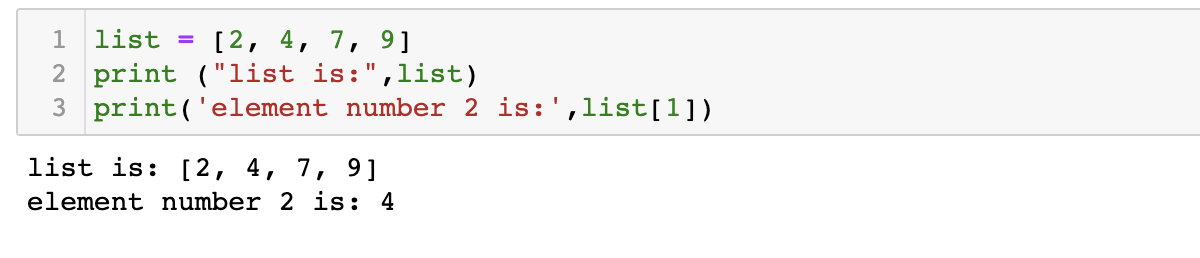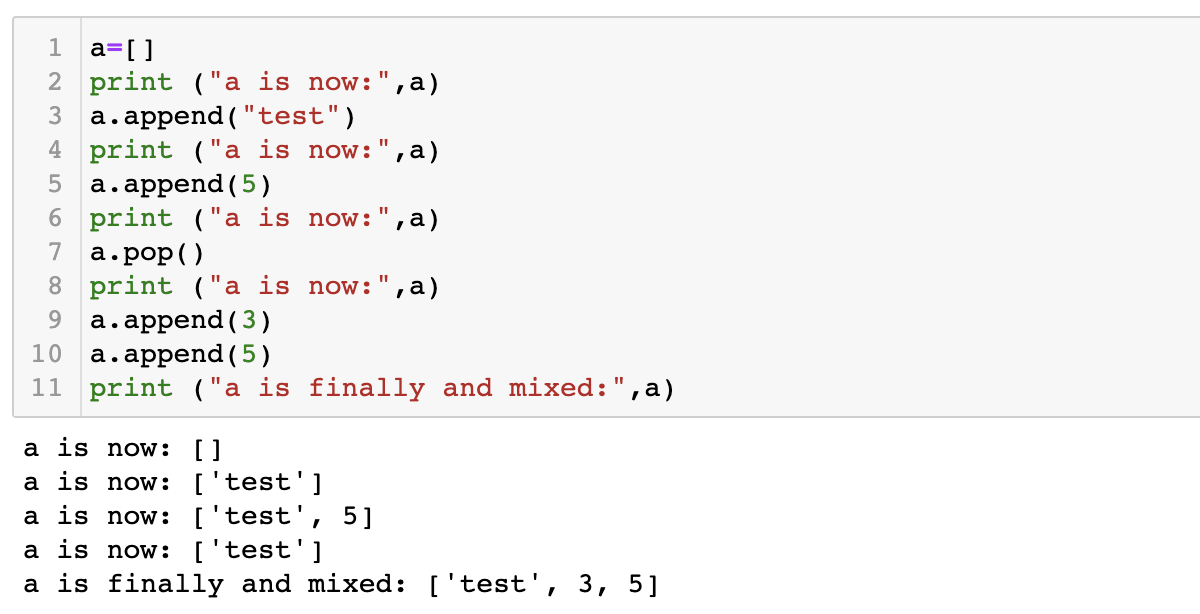# Lists

Lists are data structures that can host a various elements of different datatypes. Below, you will find the syntax for storing, accessing and modifying data that is stored in the format of a list.

## List with the same datatype:

In this example we define a list and access its elements by using their numerical position indicator. ``refers to the second element (this is because python using zero-based numbering).

```list = [2, 4, 7, 9]
print ("list is:",list)
print('element number 2 is:',list)```## List with different datatypes:

We define an array with multiple datatypes then access each element separately. The syntax is nearly identical, except for strings being contained in a pair of quotes.

```array2 = [3, "test", True, 7.4]
print ("list2 is:",array2)
print('element 1 is',array2, 'which is an integer')
print('element 2 is',array2, 'which is a string')
print('element 3 is',array2, 'which is a Boolean')
print('element 4 is',array2, 'which is a float')```

##Lists defined with the range function:

The range function can be used to define a list. The syntax of range is `range(start, finish, increment) ` If the starting point is not defined, 0 is taken as the default. If the increments are not specified, the default is units of one. The endpoint must be provided.

```a = range(5) #a = [0,1,2,3,4]
print ("a:",a)
print(a)```
```a = range(10,0,-2) #a = [10,8,6,4,2]
print ("a is now:",a)
print (a)```

##Modifying elements in an array:

Using the append and pop functions, elements can be added and removed, respectively.

```a=[]
print ("a is now:",a)
a.append("test")
print ("a is now:",a)
a.append(5)
print ("a is now:",a)
a.pop()
print ("a is now:",a)
a.append(3)
a.append(5)
print ("a is finally and mixed:",a)```